Investing Specialists

# Visualizing Option Opportunities

Get a rich depiction of option values and potential payoffs with a probability distribution.

Visualizing information, one of Morningstar's core strengths and missions, is particularly important when it comes to options. Option investing involves distilling oceans of data to gain insights about trends and valuation, and the human brain is far better at digesting volumes of information graphically than one piece at a time.

To me, the reams of option data are near-unintelligible without the intuition brought by a graphical analysis of multiple option data points. I am forever struggling to integrate all the data I can into one framework, so I can put my visual processor to work on it. Because this is the way I think about options, and I've found this technique to resonate so well with other investors, I'll graphically present option data whenever possible.

The Payoff Diagram
The payoff diagram is the graphical option analysis I most commonly see, and it's also the simplest. Most introductory option education is based around the understanding of this depiction.

The payoff diagram shows how the value of an option position varies with the price of the underlying stock. A payoff diagram is generated by plotting the value of an option position at expiration versus the stock price at expiration. An example is shown below: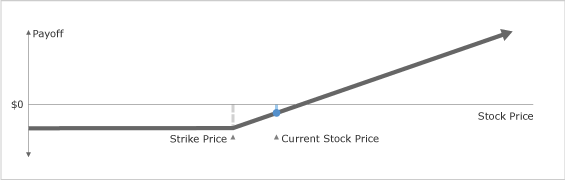While the payoff diagram is a useful first step in understanding options, it isn't really helpful for valuing options, because it doesn't communicate anything about the relative price of the options, or the likelihood of the options paying off.

Probability Distributions
I find another graphic much more useful for understanding the risk and reward tradeoffs in the options on a stock. The graphical presentation I like to use displays the probabilities of the different possible outcomes, also known as a "probability distribution."

Let me pause here for a second. Getting through this article may require your full attention, and maybe even a second reading, but developing an intuition about the probability distribution is the key to really understanding options as investments, so bear with me. It will be worth the effort.

Statistics have become so much a part of popular culture that most investors, even those without a mathematical background, have heard of the concept of a probability distribution, the most common and popular of which is the normal distribution, or "bell curve."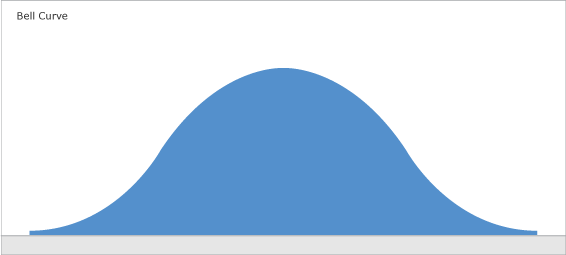The bell curve is a graphical depiction of the probability of potential outcomes for a variable. For example, the weight of Americans likely falls on a bell curve. The x axis of the graph represents weight and the Y axis of the graph represents the likelihood that an American weighs in at a given weight. The probabilities shown on the curve total 100%. The bell curve, or "normal distribution," is the mathematical model or probability distribution that fits most commonly in nature. However, the finance world tends to generate some different distribution shapes, which we'll discuss in later articles.

For now, we're hoping to build some intuition about probability distributions. So, let's use a simple example. (Like I said, bear with me. The framework becomes intuitive once it "clicks.")

If you roll a pair of dice, the outcome can range from 2 to 12, and the likelihood of each potential outcome is well understood by statisticians (assuming they're not loaded dice):Roll Outcome 2 3 4 5 6 7 8 9 10 11 12 SumProbability (%) 2.78 5.56 8.33 11.11 13.89 16.67 13.89 11.11 8.33 5.56 2.78 100If we roll the dice a very large number of times, say 1,000,000 times, we should get snake eyes (a 2) 27,800 times, a 3 55,600 times, etc. However, it is key to remember that any given roll can turn up any number, and that it takes a large number of rolls for the pattern to emerge clearly. We also note that adding all the probabilities sums to 1--every roll of the dice produces one and only one of these outcomes.

Expected Value
If we plot the outcomes in the table above on the X axis and the probabilities on the Y axis, we get a probability distribution for the outcome of the rolls of two six-sided die. Each number on the X axis is a potential outcome for the sum of the values of the two dice. The height of each bar in the histogram is equal to the probability that a pair of six-sided dice will come up with the number.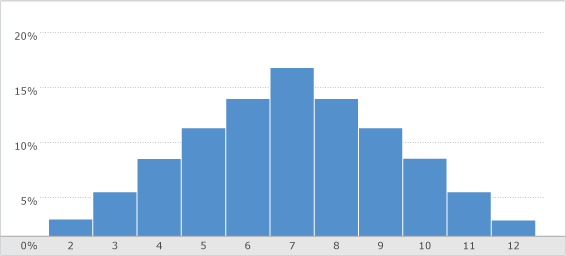Let's play with the math of this distribution a bit. If we multiply each probability times its outcome, and sum them up, we get the "expected value," or the average value we'd get for a large number of rolls. Graphically, you can visualize the expected value as the "center of gravity," or the point at which a distribution, made out of a piece of wood, would balance on a knife edge. We can add a row to our table of outcomes and calculate the expected value as follows:Roll Outcome 2 3 4 5 6 7 8 9 10 11 12 SumProbability (%) 2.78 5.56 8.33 11.11 13.89 16.67 13.89 11.11 8.33 5.56 2.78 100Probability * Outcome 0.056 0.167 0.333 0.556 0.833 1.167 1.111 1.000 0.833 0.612 0.334 7.00In this case, the expected value is 7. The expected value can also be thought of as the value of a "fair bet." Let's say you could get paid one dollar for every point that came up on a roll of a pair of fair dice, so a roll of a 4 would pay you \$4 or a roll of an 11 would pay you \$11. If you could make as many bets as you wanted, and had enough money to keep making bets forever regardless of the outcome, you would be willing to pay \$7 for that bet. But if you could buy that bet for \$6 and keep making those bets, you could quickly generate a fortune (that's how casinos make their money).

Options and Expected Payoff
At this point, you're probably thinking, "so what does all this have to do with options?"

Well, this simple graphic demonstrates the key intuition for options. Let's figure out the value of an option on a roll of these dice. Say you could buy a call option on the bet with a strike price of \$8. In other words, you'd get paid the difference between the outcome of the roll and 8 if the roll is over 8, but nothing if the roll is 8 or below. How much would you be willing to pay for that bet?

Well, you'd make \$1 if you roll a 9, \$2 if you roll a 10, etc., and the probabilities of each outcome don't change, so you can just multiply the payoff for your call option with a strike price of \$8 times the probabilities and add them up to get the expected value for the bet--\$0.56:Roll Outcome 2 3 4 5 6 7 8 9 10 11 12 SumProbability (%) 3 6 8 11 14 17 14 11 8 6 3 100Call Value (\$) 0 0 0 0 0 0 0 1 2 3 4Probability * Call Value (\$) 0 0 0 0 0 0 0 0.111 0.167 0.167 0.111 0.556We're still dealing with an ugly table of numbers, but here is where the power of the probability distribution comes in: You can represent this option graphically. If we split off the 9, 10, 11, and 12 values of the histogram, and plot them separately, we can see the expected value as the "center of gravity" of the distribution relative to the "strike price" of 8.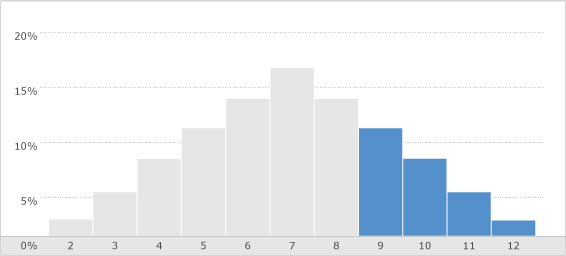By highlighting the upper tail of the probability distribution, we get a visual depiction of the value of the option, and the probabilities that it will pay off. In effect, we've "bought the right to the upside" above \$8. The area under that curve is the visual representation of the value of the option.

Now, if we could buy that right for \$0.25, it'd be a risky bet, with only a 28% (11%+8%+6%+3%) chance of paying off, but it would also be a positive expected value, with an expected payoff more than double what we'd paid for the bet (\$0.556 / \$0.25 = 2.2 times). ... And that is exactly how we'll be looking at the option investing universe going forward!

We will evaluate option investment opportunities, however probable or improbable, based upon their expected payoff relative to our estimate of the expected payoff. Remember, the difference between investing and speculation or gambling is a positive expected value. However, we'll remain very cognizant of the chance of a payoff. (How to manage the size of our investment depending on the chance of a payoff is a different question for another article.)

Bringing this back to the real world of stocks and options, if we examine a probability distribution for a stock price over, let's say, a three-year horizon, and depict a call payoff on that diagram, it would look something like the graphic below: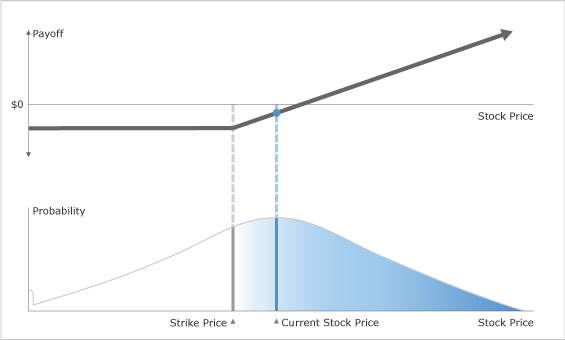The stock can trade at any price down to the penny, so the distribution will look very smooth relative to our dice example. The probability distribution for a particular stock typically centers around the current stock price and trails off in both directions. Also, any stock has a chance of bankruptcy, so there is a little jump in the probability near zero, but the price can't go below zero, so the distribution stops there.

By coloring a part of the distribution, we can see that we're "buying the upside" from the strike price by buying a call. We can also see how the option is valued (roughly) by eyeballing the center of gravity for the call area, and we can estimate the likelihood of a payoff by comparing the area under our call option with the area of the total distribution.

In the image above, the payoff diagram is included for reference, showing how buying a call has a negative return unless the stock finishes far enough in the money to offset the price of the call.  As you can see, the shaded area in the probability distribution is a much richer representation of the option than the payoff diagram, communicating option value and the relative probability of a range of different payoffs. Also, we'll be using shading to depict the slope of the line of the payoff diagram.

In later articles, we'll expand this methodology for envisioning option opportunities on the probability distribution, but the real beauty of depicting option investments this way is that all of that weird option-strategy lingo--such as "straddle," "vertical spread," and "iron condor"--can be shown as slices of the probability distribution. I'll do my best to stay away from option jargon and keep us anchored on the probability distribution by discussing how to buy or sell a bullish or bearish position by buying or selling a given piece of the probability distribution, and including a graphic for reference.

I realize this is a lot to digest.  For option neophytes, the concepts may be overwhelming, and for seasoned option investors, the concepts are just different enough to be slightly confusing. Just remember, you are looking to buy or sell pieces of a probability distribution, and you are looking for pieces of the probability distribution that the option market thinks are more or less likely to occur than you do, based on your fundamental research.